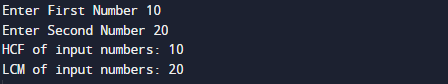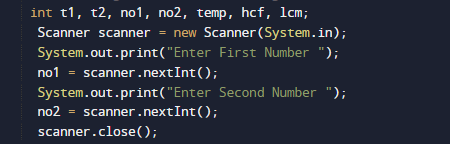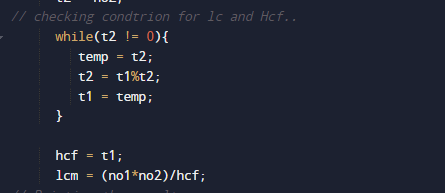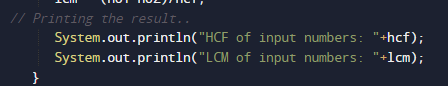# Java Program to Find HCF and LCM of Two Numbers

In this tutorial you will learn about the Java Program to Find HCF and LCM of Two Numbers and its application with practical example.

In this tutorial, we will learn to create a Java Program to Find HCF and LCM of Two Numbers using Java programming.

## Prerequisites

Before starting with this tutorial we assume that you are best aware of the following Java programming topics:

• Java Operators.
• Basic Input and Output function in Java.
• Class and Object in Java.
• Basic Java programming.
• If-else statements in Java.
• For loop in Java.

## What is LCM Number.

LCM is a method to finding the smallest possible multiplication of two or more numbers. LCM of a numbers is divisible by both or more numbers. For example, LCM of 5 ,4 and 20 is 20.

## What is HCF Number.

GCD(Greatest Common Divisor) or HCF (Highest Common Factor) . When  we finding the factor of two numbers, Highest Common Factor of  numbers is the Largest factor that divides these two numbers. The greatest factor found from the common factors is called the HCF. It is also called the Greatest common factor.

## Java Program to Find HCF and LCM of Two Numbers.

In this program we would find HCF and LCM of given numbers  .first of we would take a value sfrom user and find then we will find HCF and LCM of given numbers.

## Output

Hcf and LcmIn the above program, we have first declared and initialized a set variables required in the program.

• no1,no2= it will hold entered numbers.
• t1and t2= it will hold temp values.
• lcm = it will hold result of lcm
• Hcf = it will hold result of hcf.

After that we take a numbers from user and find HCF and LCM of given numbers.after that we calculate hcf and lcm of given number as shown in image belowAnd finally we will print both hcd and lcm of given numbers.In this tutorial we have learn about the Java Program to Find HCF and LCM of Two Numbers and its application with practical example. I hope you will like this tutorial.